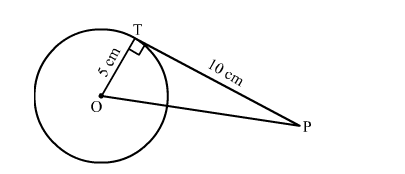# The length of the tangent from an external point P to a circle of radius 5 cm is 10 cm.`
Question:

The length of the tangent from an external point P to a circle of radius 5 cm is 10 cm. The distance of the point from the centre of the circle is
(a) 8 cm

(b) $\sqrt{104} \mathrm{~cm}$

(c) 12 cm

(d) $\sqrt{125} \mathrm{~cm}$

Solution:We know that the radius and tangent are perperpendular at their point of contact
In right triangle PTO
By using Pythagoras theorem, we have
PO2 = OT2 + TP2
⇒ PO2 = 52 + 102
⇒ PO2 = 25 + 100
⇒ PO2 = 125

$\Rightarrow \mathrm{PO}=\sqrt{125} \mathrm{~cm}$

Hence, the correct answer is option (d).